# Longest palindromic subsequence

Not to be confused with Longest palindromic substring.

The longest palindromic subsequence (LPS) problem is the problem of finding the longest subsequence of a string (a subsequence is obtained by deleting some of the characters from a string without reordering the remaining characters) which is also a palindrome. In general, the longest palindromic subsequence is not unique. For example, the string alfalfa has four palindromic subsequences of length 5: alala, afafa, alfla, and aflfa. However, it does not have any palindromic subsequences longer than five characters. Therefore all four are considered longest palindromic subsequences of alfalfa.

## Precise statement

Three variations of this problem may be distinguished:

• Find the maximum possible length for a palindromic subsequence.
• Find some palindromic subsequence of maximal length.
• Find all longest palindromic subsequences.

Theorem: Returning all longest palindromic subsequences cannot be accomplished in worst-case polynomial time.

Proof: Consider a string made up of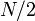$N/2$ ones, followed by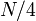$N/4$ zeroes, and finally$N/4$ ones. (Assume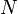$N$ is a multiple of 4, although it does not really matter.) Any palindromic subsequence either does not contain any zeroes, in which case its length is only up to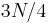$3N/4$, or it contains at least one zero. If it contains at least one zero, it must be of the form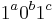$1^a0^b1^c$, but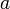$a$ and$c$ must be equal. (This is because the middle of the palindrome must lie somewhere within the zeroes, otherwise there would be no zeroes on one side of it and at least one zero on the other side; but as long as the middle lies within the zeroes, there must be an equal number of ones on each side.) But$c$ can only be up to$N/4$, and likewise with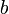$b$, so again the palindrome cannot be longer than$3N/4$ characters. However, there are$\binom{N/2}{N/4}+1$ palindromic subsequences of length$3N/4$; we can either take all the ones, or we can take all$N/4$ zeroes, all$N/4$ terminal ones, and$N/4$ out of the$N/2$ initial ones. Thus the output size is not polynomial in$N$, and then neither can the algorithm be in the worst case.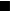$_\blacksquare$

However, this does not rule out the existence of a polynomial-time algorithm for the first two variations on the problem. We now present such an algorithm.

## Algorithm

### LCS-based approach

The standard algorithm for computing a longest palindromic subsequence of a given string S involves first computing a longest common subsequence (LCS) of S and its reverse S'. Often, this gives a correct LPS right away. For example, the reader may verify that alala, afafa, aflfa and alfla are all LCSes of alfalfa and its reverse aflafla. However, this is not always the case; for example, afala and alafa are also LCSes of alfalfa and its reverse, yet neither is palindromic. While it is clear that any LPS of a string is an LCS of the string and its reverse, the converse is false.

However, with a slight modification, this algorithm can be made to work. We will use the example string ABCDEBCA, which has six LPSes: ABCBA, ABDBA, ABEBA, ACDCA, ACECA and ACBCA (and our objective is to find one of them). Suppose that we find an LCS which is not one of these, such as L = ABDCA:

ABCDEBCA
ACBEDCBA

Now consider the central character C = D of L. It splits S up into two parts, the part before and the part after: S1 = ABC and S2 = EBCA. We know that the first half of L (L1 = AB) is a subsequence of S1, and that the second half of L (L2 = CA) is a subsequence of S2. Notice that S' is also split up into two parts: S'1 = ACBE = (S2)' and S'2 = CBA = (S1)'. Since L is also a subsequence of S', we see that L1 is a subsequence of S'1, and L2 is a subsequence of S'2. But the fact that L1 is a subsequence of S'1 implies (by reversing both strings) that (L1)' = BA is a subsequence of S2 = EBCA. Since L1 is a subsequence of S1 and (L1)' is a subsequence of S2, we conclude that L1C(L1)' = ABDBA is a subsequence of S1CS2 = S. So we have obtained the desired result, a longest palindromic subsequence.

Extrapolating to the general case, we can always obtain a LPS by first taking the LCS of S and S' and then "reflecting" the first half of the result onto the second half; that is, if L has k characters, then we replace the last ⌊k/2⌋ characters of L by the reverse of the first ⌊k/2⌋ characters of L to obtain a palindromic subsequence of S. This is obviously a palindrome by the foregoing analysis; it is guaranteed to be a subsequence by the foregoing analysis; and it is guaranteed to be as long as possible because it is of the same length as any LCS, yet the length of an LCS is also the upper bound on the length of an LPS since every LPS must trivially be an LCS. The odd case is handled as above, and the even case very similarly (lacking only the central character C).

The complexity of this algorithm is the same as the complexity of the LCS algorithm used. The textbook dynamic programming algorithm for LCS runs in$O(mn)$ time, so the time taken to find the LPS is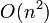$O(n^2)$, where$n$ is the length of S.

### Direct solution

There is also a "direct"$O(n^2)$ solution, also based on dynamic programming; it is very similar in principle to the approach based on the textbook LCS algorithm. We define$f(i,j)$ to be the length of the longest palindromic subsequence of the substring S[i,j) (see half-open interval). Assuming indexing starting from zero, the objective is to compute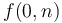$f(0,n)$.

This is easy when the substring is empty, or when it consists of only a single character; the value of$f$ will be 0 or 1, respectively. Otherwise,

• when the substring's first and last characters are equal, add both of them to the longest palindromic subsequence of the characters in between to get a palindromic subsequence two characters longer;
• when they are unequal, then it's clearly not possible to use both of them to form a palindromic subsequence of the given substring, so the answer will be the same as if one of them were ignored.

Thus:$f(i,j) = \begin{cases} 0 & \text{if } j-i = 0 \\ 1 & \text{if } j-i = 1 \\ \max(f(i+1,j),f(i,j-1)) & \text{if } j-i > 1 \text{ and } S_i \neq S_{j-1} \\ 2 + f(i+1,j-1) & \text{if } j-i > 1 \text{ and } S_i = S_{j-1} \end{cases}$

To see calculate the cost, consider that the worst case occurs when every invocation to$f$ requires a call on two different substrings. This can happen for invocations with strings of length$n$ to$2$. So the total number of invocations is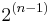$2^{(n-1)}$.

However, a lot of these invocations are repeats. If we store previously computed solutions, then the cost goes down. The above worst case happens when no matches are ever made, so this requires us to try out eliminating all strings that start at 0 and those that start at n-1, such that their combined length is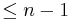$\leq n-1$. This is just the number of ways to partition a given number into two non-negative integers, summed up over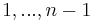$1 ,..., n-1$. The number of ways to partition$k$ into two non-negative integers is$k + 1$. Hence the overall cost is$O(n^2)$.